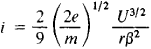# Langmuir Formula

The following article is from The Great Soviet Encyclopedia (1979). It might be outdated or ideologically biased.

## Langmuir Formula

(three-halves power law), the dependence of the electric current between two electrodes (a cathode and an anode) in a vacuum on the potential difference U between them. Such a current is usually carried by electrons (although the Langmuir formula is also suitable, in a somewhat altered form, for ionic currents). Electrons are emitted from the cathode as a result of thermionic emission or electron emission of another type. The action of the restraining field of the space charge formed between the electrodes by the aggregate of the electrons already there counteracts the electric field deflecting the electrons toward the anode. This “electron cloud” impedes the motion of electrons newly ejected from the cathode. The specific form of the Langmuir formula depends on the shape of the electrodes and the geometry of the interelectrode space, but for all simple geometries (and in a number of more complex configurations) it follows from this formula that the current is proportional to U3/2 (hence the name “three-halves power law”).

The Langmuir formula was first derived for the particular case of infinitely extended flat electrodes by the American physicist C. D. Child in 1911, on the simplifying assumption that the initial velocities of the electrodes leaving the cathode are equal to zero. However, it was named for I. Langmuir, who in 1913 studied the relation for other electrode configurations. For example, the formula derived by Langmuir has the following form for coaxial cylindrical electrodes from which theinner electrode emits electrons:where i is the current per unit length of the cylinders, e and m are the charge and mass of an electron, and β is some function of the ratio of the radiuses of the inner and outer cylinders r and r0. The relation reflected by the Langmuir formula has been studied—taking into account the nonzero initial velocities of the electrons—both by Langmuir (1923) and by other physicists, in particular W. Schottky (1914) and S. A. Boguslavskii (1923), who was the first to calculate accurately the values of the function β. Analysis of the Langmuir formula shows that the minimum potential (the area within which the potential is less than the cathode’s potential) arises in the interelectrode space.

The Langmuir formula is valid for values of U for which not all electrons emerging from the cathode reach the anode—that is, only for currents weaker than the saturation current. The formula plays an important role in the design and construction of vacuum electronic devices, particularly hot-cathode tubes.

### REFERENCES

Dobretsov, L. N. Elektronnaia i ionnaia emissiia. Moscow-Leningrad, 1952.
Gaponov, V. I. Elektronika, part 1. Moscow, 1960.